Test: Fluid Mechanics Level - 3

# Test: Fluid Mechanics Level - 3

Test Description

## 25 Questions MCQ Test Mechanical Engineering SSC JE (Technical) | Test: Fluid Mechanics Level - 3

Test: Fluid Mechanics Level - 3 for Mechanical Engineering 2023 is part of Mechanical Engineering SSC JE (Technical) preparation. The Test: Fluid Mechanics Level - 3 questions and answers have been prepared according to the Mechanical Engineering exam syllabus.The Test: Fluid Mechanics Level - 3 MCQs are made for Mechanical Engineering 2023 Exam. Find important definitions, questions, notes, meanings, examples, exercises, MCQs and online tests for Test: Fluid Mechanics Level - 3 below.
Solutions of Test: Fluid Mechanics Level - 3 questions in English are available as part of our Mechanical Engineering SSC JE (Technical) for Mechanical Engineering & Test: Fluid Mechanics Level - 3 solutions in Hindi for Mechanical Engineering SSC JE (Technical) course. Download more important topics, notes, lectures and mock test series for Mechanical Engineering Exam by signing up for free. Attempt Test: Fluid Mechanics Level - 3 | 25 questions in 50 minutes | Mock test for Mechanical Engineering preparation | Free important questions MCQ to study Mechanical Engineering SSC JE (Technical) for Mechanical Engineering Exam | Download free PDF with solutions
 1 Crore+ students have signed up on EduRev. Have you?
Test: Fluid Mechanics Level - 3 - Question 1

### If cohesion between molecules of a fluid is greater than adhesion between fluid and glass, then the free level of fluid in a dipped glass tube will be

Test: Fluid Mechanics Level - 3 - Question 2

### An open vessel of water is accelerated up an inclined plane. The free water surface will

Test: Fluid Mechanics Level - 3 - Question 3

### The line of action of the buoyant force acts through the centroid of the

Test: Fluid Mechanics Level - 3 - Question 4

The time oscillation of a floating body with increase in metacentric height will be

Test: Fluid Mechanics Level - 3 - Question 5

The velocity at the exit of the pipe as shown in the below figure will be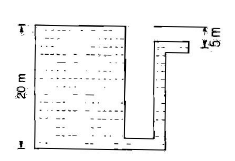Test: Fluid Mechanics Level - 3 - Question 6

The following terms relate to floating bodies :
Centre of gravity .....G
Meta Centre .....M
Weight of floating body.....W
Buoyant force ......FB
Match List I with List II and select these correct answer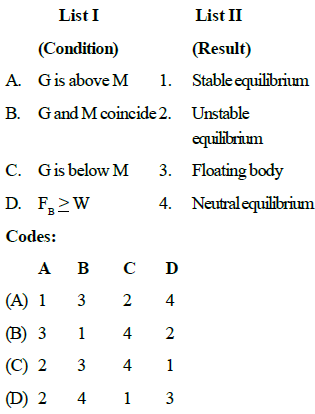Test: Fluid Mechanics Level - 3 - Question 7

Match List I with List II and select the correct answer.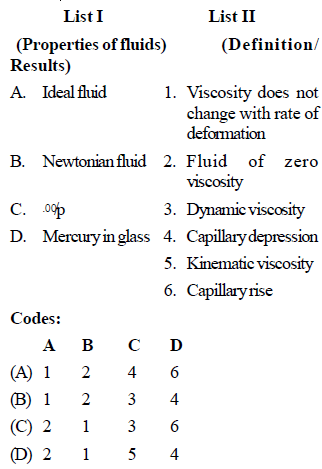Detailed Solution for Test: Fluid Mechanics Level - 3 - Question 7

Explanation : a) A fluid that has no resistance to shear stress is known as an ideal or inviscid fluid. Zero viscosity is observed only at very low temperatures in superfluids. Otherwise, the second law of thermodynamics requires all fluids to have positive viscosity; such fluids are technically said to be viscous or viscid.

A Newtonian fluid is one whose viscosity is not affected by shear rate: all else being equal, flow speeds or shear rates do not change the viscosity.

The liquid which wets the wall of tube rises in the tube and the liquid which does not wet the wall of the tube descends in the tube. For example, when a glass capillary tube is dipped in water, water rises in the tube and the shape of water meniscus in concave, similarly when a glass capillary tube is dipped into mercury; mercury descends in the tube and the shape of mercury meniscus is convex.

Test: Fluid Mechanics Level - 3 - Question 8

Match List I with List II and select the correct answer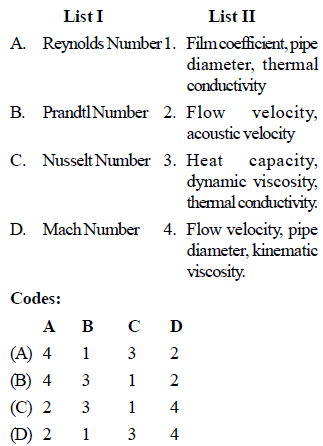Test: Fluid Mechanics Level - 3 - Question 9

Match List I (fluid properties) with List II (related terms) and select the correct answer.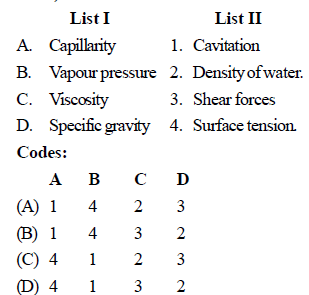Test: Fluid Mechanics Level - 3 - Question 10

Match List I with List II and select the correct answer.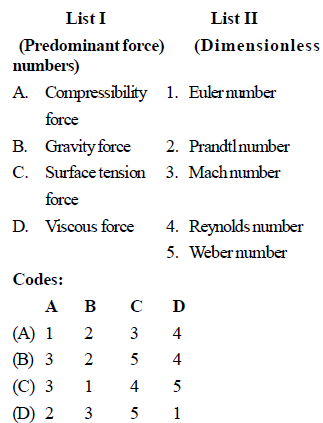Test: Fluid Mechanics Level - 3 - Question 11

Newton's law of viscosity for a fluid states that the shear stress is

Test: Fluid Mechanics Level - 3 - Question 12

A vertically immersed surface is shown in the below figure. The distance of its centre of pressure from the water surface is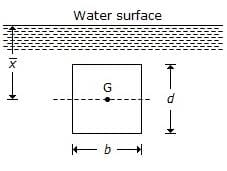Test: Fluid Mechanics Level - 3 - Question 13

If angle of contact of a drop of liquid is acute than

Test: Fluid Mechanics Level - 3 - Question 14

Match List I (Physical properties of fluid) with List II (Dimensions/definitions) and select the correct answer using the codes given below the lists: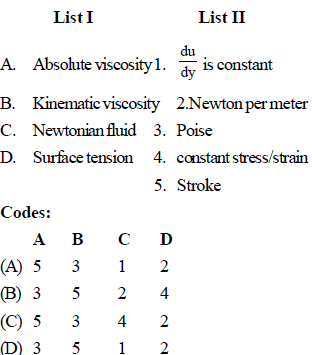Test: Fluid Mechanics Level - 3 - Question 15

An increase in pressure of 2 bars decreases the volume of a liquid by 0.01 percent. The bulk modulus of elasticity of the liquid is

Test: Fluid Mechanics Level - 3 - Question 16

The pressure inside a soap bubble of 50 mm diameter is 25 N/m2 above the atmospheric pressure. What is the surface tension is soap film

Test: Fluid Mechanics Level - 3 - Question 17

Bourdon gauge measures

Test: Fluid Mechanics Level - 3 - Question 18

When can a piezometer be not used for pressure measurement in pipes

Test: Fluid Mechanics Level - 3 - Question 19

How is the difference of pressure head h measured by mercury oil differential manometer expressed

where × = Manometer reading Sg and So are the specific gravities of mercury and oil respectively

Test: Fluid Mechanics Level - 3 - Question 20

In order to increase sensitivity of U-tube manometer, one leg is usually inclined by angle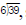sensitivity of inclined tube to sensitivity of Utube equal to

Test: Fluid Mechanics Level - 3 - Question 21

In figure if the pressure of gas in bulb A is 50 cm Hg vacuum and Patm = 76 cm Hg, the height of column H is equal to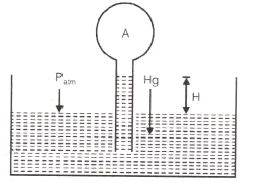Test: Fluid Mechanics Level - 3 - Question 22

A U-tube manometer is used to measure the pressure in an oil pipe A as shown in the figure. The specific gravity of the oil is 0.8 and that of mercury is 13.6. The equivalent gauge pressure is nearly.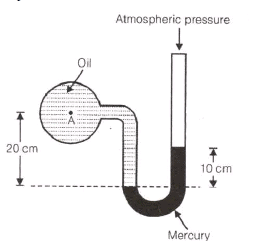Test: Fluid Mechanics Level - 3 - Question 23

A multi-tube manometer filled with water up to level A, B and C as shown in the figure is rotated about the vertical axis at A. The water levels at A, B and C will all lie on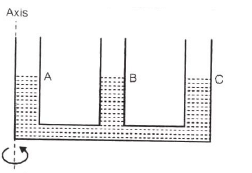Test: Fluid Mechanics Level - 3 - Question 24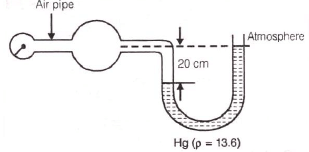A U-tube manometer shown attached to an air pipe reads a height of 20 cm of mercury as shown. What is the pressure in kPa in the air pipe ?

Test: Fluid Mechanics Level - 3 - Question 25

A metal block is thrown into deep lake. As it sinks deeper in water, the buoyant force acting on it

## Mechanical Engineering SSC JE (Technical)

6 videos|97 docs|55 tests
 Use Code STAYHOME200 and get INR 200 additional OFF Use Coupon Code
Information about Test: Fluid Mechanics Level - 3 Page
In this test you can find the Exam questions for Test: Fluid Mechanics Level - 3 solved & explained in the simplest way possible. Besides giving Questions and answers for Test: Fluid Mechanics Level - 3, EduRev gives you an ample number of Online tests for practice

## Mechanical Engineering SSC JE (Technical)

6 videos|97 docs|55 tests